Courses

# Chapter Notes - Mensuration Class 6 Notes | EduRev

## Class 6 : Chapter Notes - Mensuration Class 6 Notes | EduRev

The document Chapter Notes - Mensuration Class 6 Notes | EduRev is a part of the Class 6 Course Mathematics (Maths) Class 6.
All you need of Class 6 at this link: Class 6

Mensuration

Mensuration is a branch of mathematics which is a topic in Geometry. It  is a study of various geometrical shapes, their length, breadth, volume, and area for 2D as  well as 3D shapes. Some important terminologies included in this topic are covered below.

Perimeter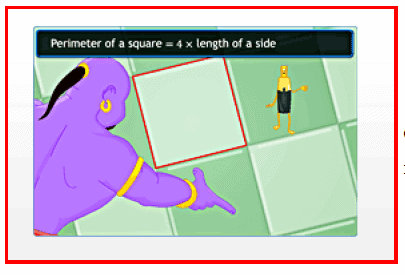Perimeter is the distance covered along the boundary forming a closed figure when we go round the figure once. The concept of perimeter is widely used in real life. For Example:

1. For fencing land.
2. For building a compound wall around a house.

Closed figure

• A figure with no open ends is a closed figure.
• Regular closed figures: A closed figure in which all the sides and angles equal.
• The perimeter of a regular closed figure is equal to the sum of its sides.

Perimeter of a rectangle:

= 2 (l + b)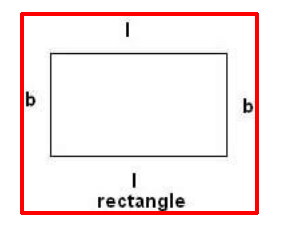Perimeter of a square:

= s+s+s+s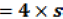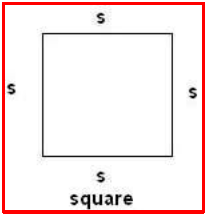Equilateral triangle:

A triangle with all its sides and angles equal is called an equilateral triangle.

The perimeter of an equilateral triangle with the side 'a'=a+a+a =3 x a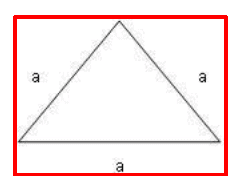Area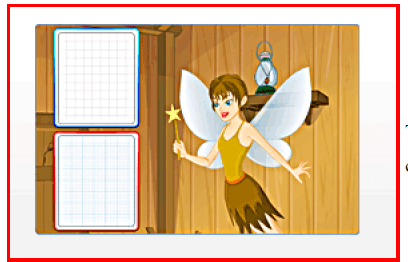The amount of surface enclosed by a closed figure is called its area. The following conventions are to be adopted while calculating the area of a closed figure using a squared or graph paper.

• Count the fully-filled squares covered by the closed figure as one square unit or unit square each.
• Count the half-filled squares as half a square unit.
• Count the squares that are more than half-filled as one square unit.
• Ignore the squares filled less than half.

For example, the area of this shape can be calculated as shown: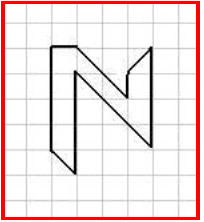Covered area Number Area estimate (sq. units) Fully filled squares 6 6 Half-filled squares 7 7 x 1/2 Squares filled more than half 0 0 Squares filled less than half 0 0
• Area covered by full squares = 6 x 1 = 6 sq. units Area covered by half squares = 7 x ½ = 7/2= 3 ½ sq. units.
• Total area of the given shape = 6 + 3 ½ sq. units Thus, the total area of the given shape = 9 ½ sq. Units.
• Area of a rectangle can be obtained by multiplying length by breadth. Area of the square can be obtained by multiplying side by side.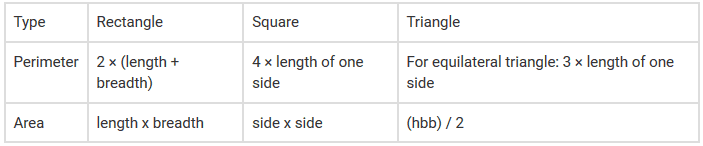Offer running on EduRev: Apply code STAYHOME200 to get INR 200 off on our premium plan EduRev Infinity!

## Mathematics (Maths) Class 6

185 videos|229 docs|43 tests

,

,

,

,

,

,

,

,

,

,

,

,

,

,

,

,

,

,

,

,

,

;